# 9.1.3. 3D and 4D niimgs: handling and visualizing¶

Here we discover how to work with 3D and 4D niimgs.

```from nilearn import datasets
print('Datasets are stored in: %r' % datasets.get_data_dirs())
```

Out:

```Datasets are stored in: ['/home/nicolas/nilearn_data']
```

Let’s now retrieve a motor contrast from a Neurovault repository

```motor_images = datasets.fetch_neurovault_motor_task()
motor_images.images
```

Out:

```['/home/nicolas/nilearn_data/neurovault/collection_658/image_10426.nii.gz']
```

motor_images is a list of filenames. We need to take the first one

```tmap_filename = motor_images.images
```

## 9.1.3.2. Visualizing a 3D file¶

The file contains a 3D volume, we can easily visualize it as a statistical map:

```from nilearn import plotting
plotting.plot_stat_map(tmap_filename)
```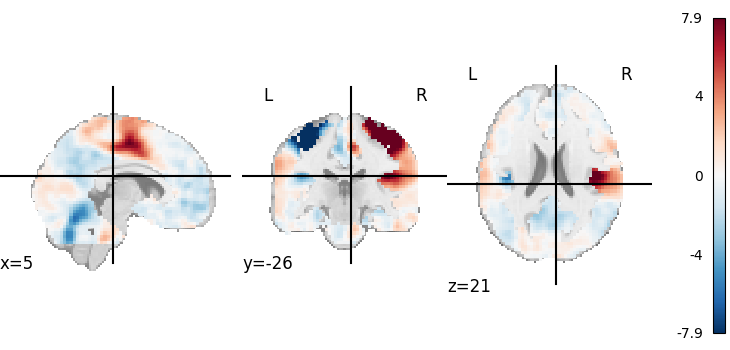Out:

```/home/nicolas/GitRepos/nilearn-fork/nilearn/plotting/img_plotting.py:300: FutureWarning: Default resolution of the MNI template will change from 2mm to 1mm in version 0.10.0

<nilearn.plotting.displays._slicers.OrthoSlicer object at 0x7fa52d3da2b0>
```

Visualizing works better with a threshold

```plotting.plot_stat_map(tmap_filename, threshold=3)
```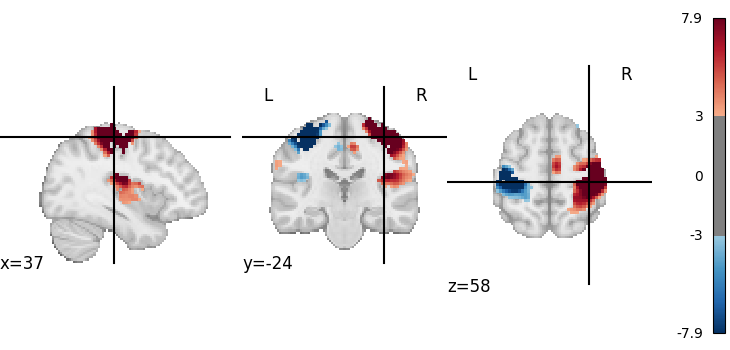Out:

```<nilearn.plotting.displays._slicers.OrthoSlicer object at 0x7fa52d4e1b20>
```

## 9.1.3.3. Visualizing one volume in a 4D file¶

```rsn = datasets.fetch_atlas_smith_2009()['rsn10']
rsn
```

Out:

```'/home/nicolas/nilearn_data/smith_2009/PNAS_Smith09_rsn10.nii.gz'
```

It is a 4D nifti file. We load it into the memory to print its shape.

```from nilearn import image
```

Out:

```(91, 109, 91, 10)
```

We can retrieve the first volume (note that Python indexing starts at 0):

```first_rsn = image.index_img(rsn, 0)
print(first_rsn.shape)
```

Out:

```(91, 109, 91)
```

first_rsn is a 3D image.

We can then plot it

```plotting.plot_stat_map(first_rsn)
```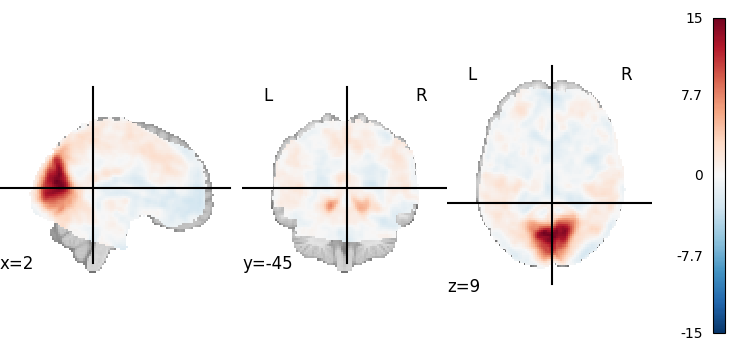Out:

```<nilearn.plotting.displays._slicers.OrthoSlicer object at 0x7fa52d336a60>
```

## 9.1.3.4. Looping on all volumes in a 4D file¶

If we want to plot all the volumes in this 4D file, we can use iter_img to loop on them.

Then we give a few arguments to plot_stat_map in order to have a more compact display.

```for img in image.iter_img(rsn):
# img is now an in-memory 3D img
plotting.plot_stat_map(img, threshold=3, display_mode="z", cut_coords=1,
colorbar=False)
```
•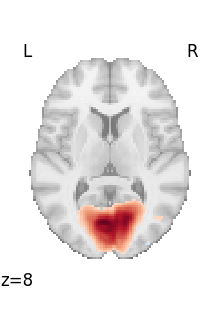•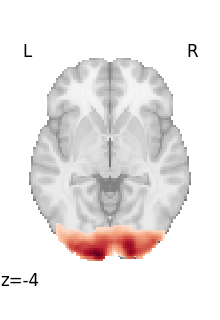•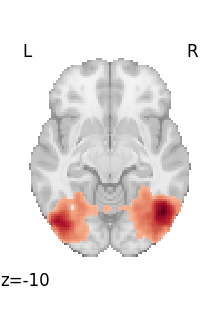•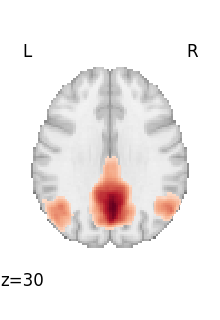•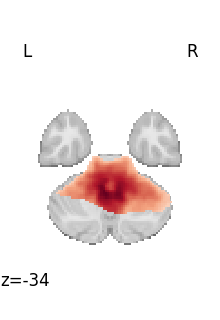••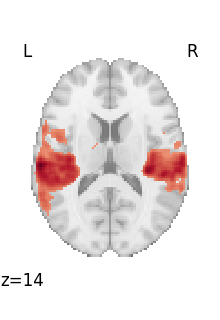•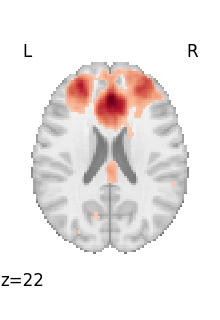•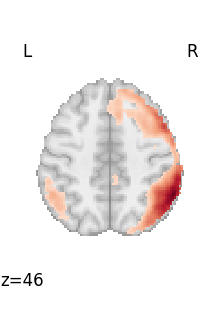•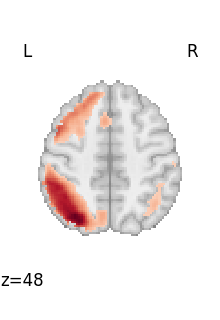## 9.1.3.5. Looping through selected volumes in a 4D file¶

If we want to plot selected volumes in this 4D file, we can use index_img with the slice constructor to select the desired volumes.

Afterwards, we’ll use iter_img to loop through them following the same formula as before.

```selected_volumes = image.index_img(rsn, slice(3, 5))
```

If you’re new to Python, one thing to note is that the slice constructor uses 0-based indexing. You can confirm this by matching these slices to the previous plot above.

```for img in image.iter_img(selected_volumes):
plotting.plot_stat_map(img)
```
•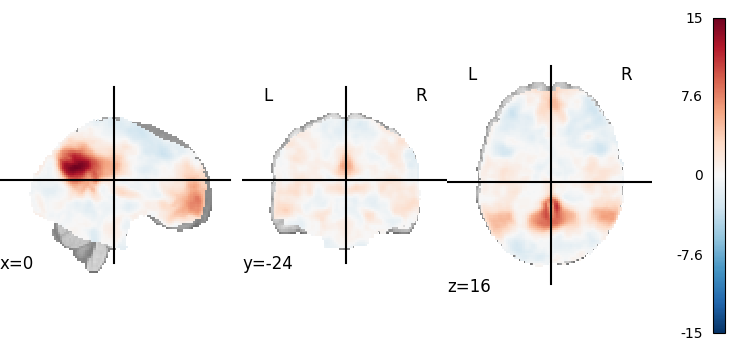•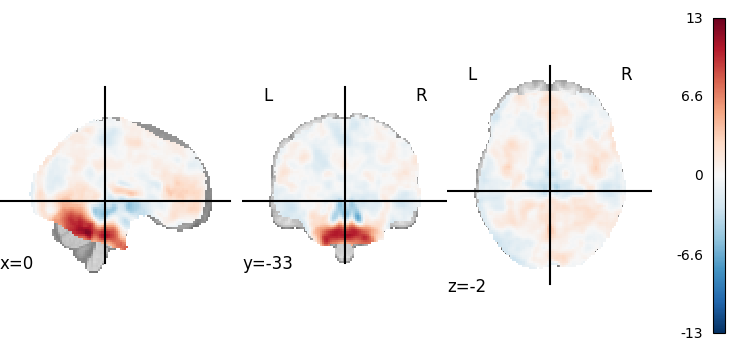plotting.show is useful to force the display of figures when running outside IPython

```plotting.show()
```

To recap, neuroimaging images (niimgs as we call them) come in different flavors:

• 3D images, containing only one brain volume

• 4D images, containing multiple brain volumes.

More details about the input formats in nilearn for 3D and 4D images is given in the documentation section: Inputing data: file names or image objects.

Functions accept either 3D or 4D images, and we need to use on the one hand `nilearn.image.index_img` or `nilearn.image.iter_img` to break down 4D images into 3D images, and on the other hand `nilearn.image.concat_imgs` to group a list of 3D images into a 4D image.

Total running time of the script: ( 0 minutes 15.346 seconds)

Estimated memory usage: 127 MB

Gallery generated by Sphinx-Gallery